## Mathematic Support of Dilation Flooding Effect

### The only verifiable cause of observed cosmic redshift.

In nearly all finite universe models, it is a requirement that all standard model particles came into existence simultaneously and over a great distance. Regardless of the model used, whether Big Bang or otherwise, the state of the matter in the universe no later than it’s first second of existence appears to be:

If we accept the theory that the standard model particles of our universe did not exist until that time, then characteristics directly attributable to those particles can also be assumed to not exist.

This is supported by the Higgs boson theory which proposes that it is through the mechanism of that particle which gives mass to other particles. If the origin of the universe was such that this particle dominated the universe and, in a very brief period, decayed into the dilation (or gravity) contributing particles that now exist, then that implies the first moments of the universe were gravity naïve.

From that time, the time dilation or “gravity waves” being emitted by all the particles in the universe have been traveling outward. Per CERN, “Gravity is the weakest [force] but it has an infinite range,” implying that even the time dilation of a photon first emitted by a Higgs boson decay 14 billion years ago is just reaching the earth now.

Although that would appear to be an infinitesimally small amount, the entire universe is simply a collection of these small amounts. But it is the cumulative effect of those tiny values to which we are interested.

If we presume that the universe is a static three-dimensional volume, we can also presume that the furthest distance from which we would receive new gravity waves would be comparable to the most distant electromagnetic signals we can observe. So for about 13.7 billion light years, new gravity has been reaching us as well as the EM evidence of these ancient signals.

Therefore, if we turn back the clock a few eons we should be seeing a shorter distance into the universe and therefore under less influence from more distant gravity as well. But is that really worth considering?

Let’s look at it as simply as possible with the following equations: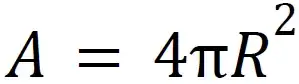##### Area of a sphere.

We will use this for calculating the quantity of particles at a distance from our point of measurement.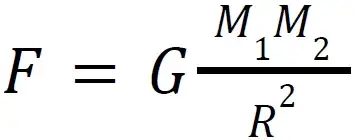##### Force of gravity between two masses

We will be calculating the gravitational force on our point of measurement (M1) in relation to all of the particles at the same spherical distance (M2).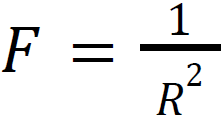##### Inverse square law

In this case, gravity is the F value, and we substitute Radius for Distance (both being linear measurement values).
This is implied by the Force of Gravity equation, but is included here to highlight that we will be seeing this effect reflected in our results.

If we assume that the particle density of the universe is and has been on average nearly uniform as implied by observation of the CMB, we can perform the following calculation to determine the gravitational influence of the matter at any distance from one point in space: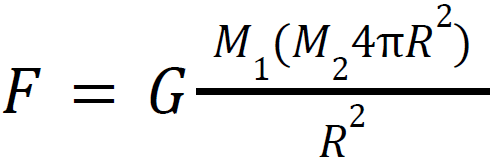M1 represents the fixed location for this observation.

M2 is all mass that is not M1

Assuming equivalent average masses at equivalent distances, then M1=M2

Since the quantity of M2 changes by distance it is multiplied by the surface area of the spherical distance from M1.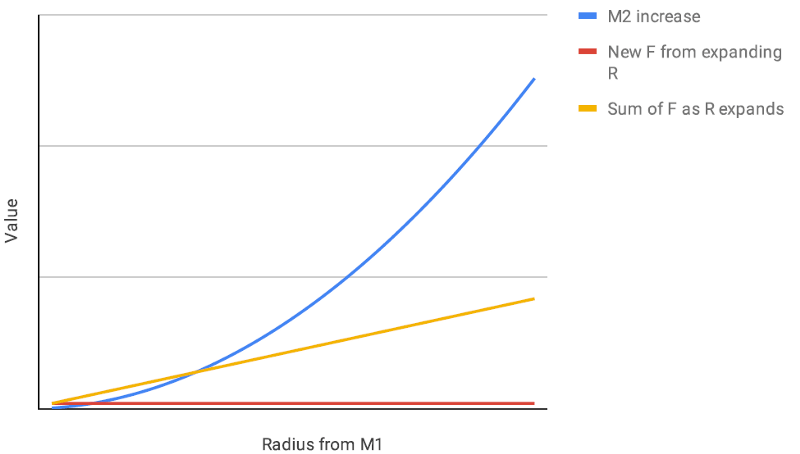If we keep the same values for M1 and M2, the solution for all values of R provide the same result. That means the gravitational influence of all matter at 14 BLY away is the same force as all matter at 1 parsec away.

This is computational proof of linear addition of gravitational force over time.

Integration of this equation for all distances shows a linear trend of gravitational force accumulation on any given point (M1 location) in the universe.

The summation of gravitational force over time matches the extant linear measurement trend of cosmic redshift.

This equation includes the constant G, so changes to M1 and M2 produce different results. Through analysis of observational data we can determine with great certainty the average standard model particle density of the universe.

Since through direct observation we cannot know with confidence the quantity and distribution of hard to detect particles and objects in the universe (like neutrinos and black holes), Dilation Flooding computation will reveal the true standard model particle density of the universe.

Using Einstein's equivalence principle, this theory clearly demonstrates through the progressive change in time dilation (gravitational potential) an alternative explanation for cosmic redshift computations.  This is an improvement over Hubble's accelerating cosmic expansion hypothesis being that such acceleration has never been proven and is becoming increasingly difficult to justify in modern observations.
Dilation Flooding is perhaps the only verifiable theory to explain the observational trend of cosmic red shift without the need for additional unknown forces or matter, and we can apply this information to answer computational puzzles like quasar energy levels and explain galactic densities.

For more elucidation on this theory, see Dilation Flooding